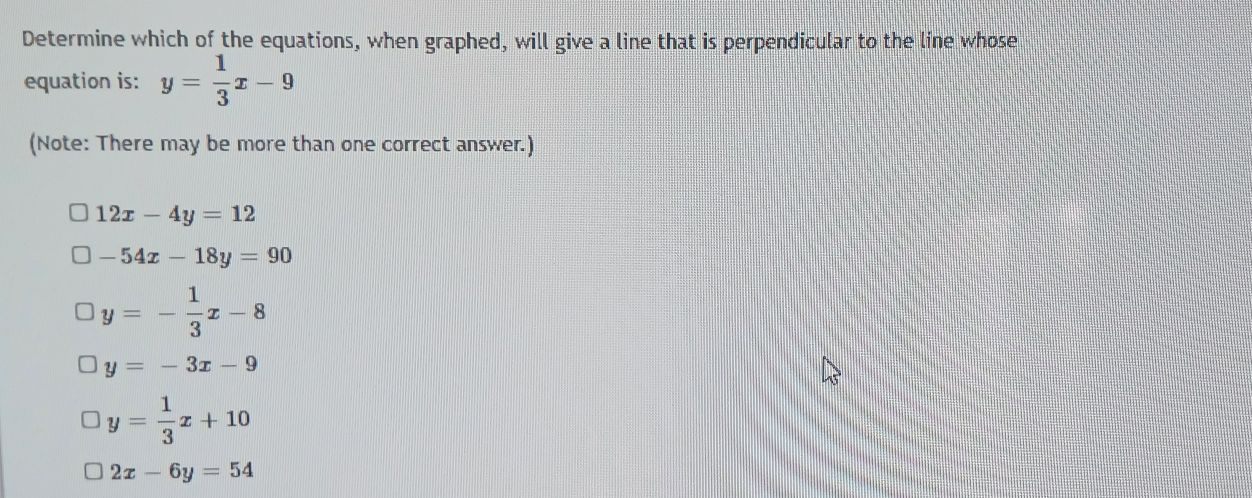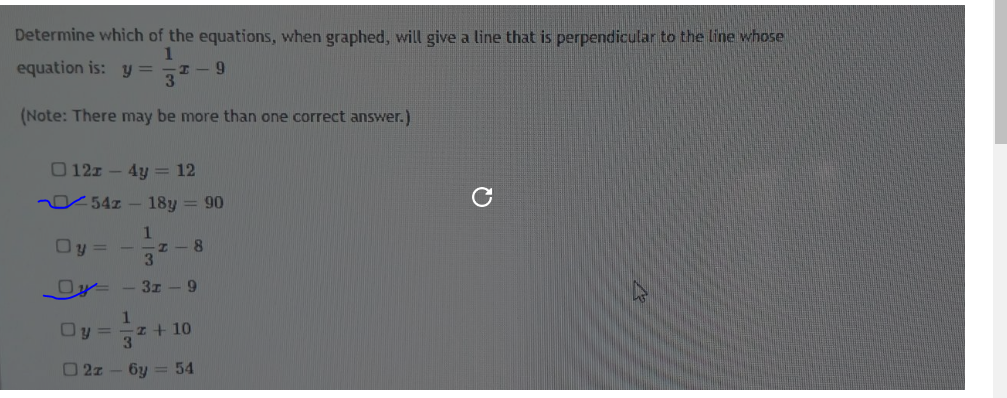### Still have math questions?

Algebra
QuestionDetermine which of the equations, when graphed, will give a line that is perpendicular to the line whose equation is: $$y = \frac { 1 } { 3 } x - 9$$

(Note: There may be more than one correct answer.)

$$12 x - 4 y = 12$$

$$- 54 x - 18 y = 90$$

$$y = - \frac { 1 } { 3 } x - 8$$

$$y = - 3 x - 9$$

$$y = \frac { 1 } { 3 } x + 10$$

$$2 x - 6 y = 54$$